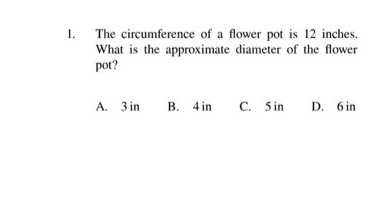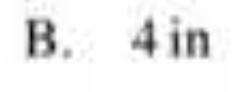### ¿Todavía tienes preguntas de matemáticas?

Pregunte a nuestros tutores expertos
Algebra
Pregunta1. The circumference of a flower pot is $$12$$ inches. What is the approximate diameter of the flower pot?

A. $$3$$ in B. $$4$$ in C. $$5$$ in D. $$6$$ in